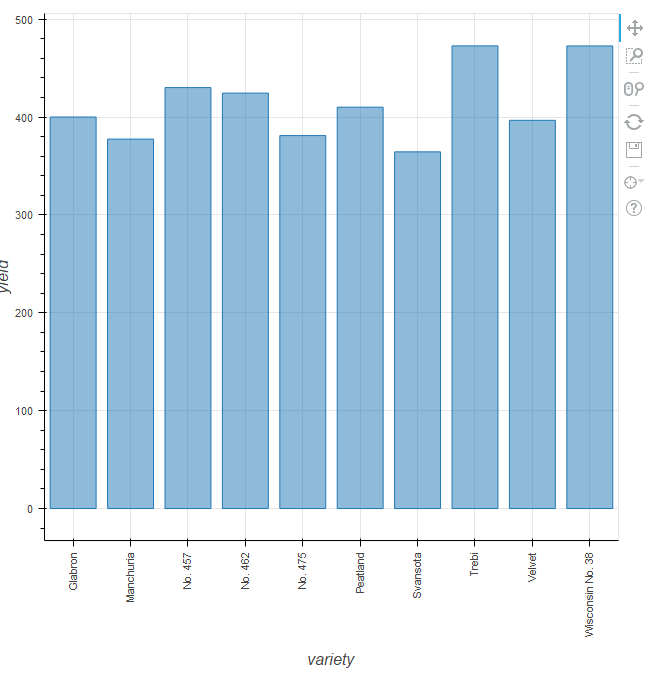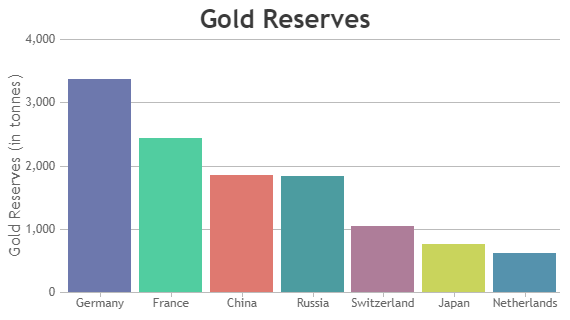# Rbokeh barplot x-axis reordered by y-axis value

Here is a simple example of barplot expressed in Rbokeh.

library(rbokeh)

# total yield per variety

figure() %>%

ly_bar(variety, yield, data = lattice::barley, hover = TRUE) %>%

theme_axis(“x”, major_label_orientation = 90)

Result are shown as belowQuestion 1)

I want to plot bars, reordered on x-axis by yield in descending order like belowI know that there’s simple way of doing this in ggplot with ‘reorder’ function, but have no idea how to do this in Rbokeh.

How can I do this?

Question 2)

Running the code above, I can see this error message, what does this mean and how can I solve this problem?

Warning messages:

1: In structure(x, class = unique(c(“AsIs”, oldClass(x)))) :

Calling ‘structure(NULL, *)’ is deprecated, as NULL cannot have attributes.

2: In structure(x, class = unique(c(“AsIs”, oldClass(x)))) :

Calling ‘structure(NULL, *)’ is deprecated, as NULL cannot have attributes.

3: In structure(x, class = unique(c(“AsIs”, oldClass(x)))) :

Calling ‘structure(NULL, *)’ is deprecated, as NULL cannot have attributes.

4: In structure(x, class = unique(c(“AsIs”, oldClass(x)))) :

Calling ‘structure(NULL, *)’ is deprecated, as NULL cannot have attributes.

5: In structure(x, class = unique(c(“AsIs”, oldClass(x)))) :

Calling ‘structure(NULL, *)’ is deprecated, as NULL cannot have attributes.

6: In structure(x, class = unique(c(“AsIs”, oldClass(x)))) :

Calling ‘structure(NULL, *)’ is deprecated, as NULL cannot have attributes.

7: In structure(x, class = unique(c(“AsIs”, oldClass(x)))) :

Calling ‘structure(NULL, *)’ is deprecated, as NULL cannot have attributes.

Hi,

Regardless of language, sorting the columns means sorting the list of factors that make up the underlying FactorRange object. Here is how you can do it in Python, e.g.

I am not sure if the list of factors is easily configurable in the current RBokeh binding. You might have better luck posing this question on the rbokeh GH issue tracker.

Thanks,

Bryan

···

On Feb 6, 2019, at 07:29, Wookeun Lee <[email protected]> wrote:

Here is a simple example of barplot expressed in Rbokeh.

library(rbokeh)

# total yield per variety
figure() %>%
ly_bar(variety, yield, data = lattice::barley, hover = TRUE) %>%
theme_axis("x", major_label_orientation = 90)

Result are shown as below

<캡처.PNG>

**Question 1)**
I want to plot bars, reordered on x-axis by yield in descending order like below

I know that there's simple way of doing this in ggplot with 'reorder' function, but have no idea how to do this in Rbokeh.

How can I do this?

**Question 2)**
Running the code above, I can see this error message, what does this mean and how can I solve this problem?

Warning messages:
1: In structure(x, class = unique(c("AsIs", oldClass(x)))) :
Calling 'structure(NULL, *)' is deprecated, as NULL cannot have attributes.
2: In structure(x, class = unique(c("AsIs", oldClass(x)))) :
Calling 'structure(NULL, *)' is deprecated, as NULL cannot have attributes.
3: In structure(x, class = unique(c("AsIs", oldClass(x)))) :
Calling 'structure(NULL, *)' is deprecated, as NULL cannot have attributes.
4: In structure(x, class = unique(c("AsIs", oldClass(x)))) :
Calling 'structure(NULL, *)' is deprecated, as NULL cannot have attributes.
5: In structure(x, class = unique(c("AsIs", oldClass(x)))) :
Calling 'structure(NULL, *)' is deprecated, as NULL cannot have attributes.
6: In structure(x, class = unique(c("AsIs", oldClass(x)))) :
Calling 'structure(NULL, *)' is deprecated, as NULL cannot have attributes.